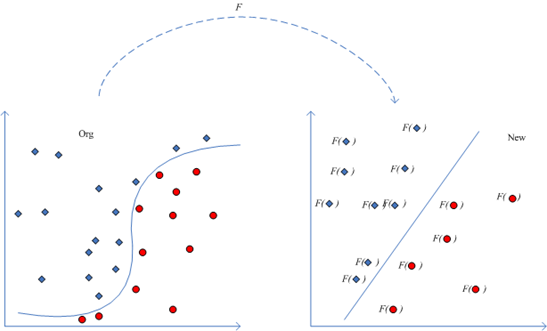# 支持向量机学习之2：核函数

Spark机器学习系列之13： 支持向量机SVM ：http://blog.csdn.net/qq_34531825/article/details/52881804

SVM最终要转换为对偶问题如下：

maxW(α)=i=1nαi12i,j=1nyiyjαiαj<xi,xj>

s.t.i=1nαiyi=0αi0i=1...n

F=keqqr2

lnF(q,q,r)=ln(ke)+ln(q)+ln(q)2ln(r)>
，令x2=ln(q)x3=ln(q)x4=ln(r)x1=in(ke)G(x⃗ )=ln(F(q,q,r))$x_2=ln(q)，x_3=ln(q^{'})，x_4=ln(r)，x_1=in(k_e)，G(\vec{x})=ln(F(q,q^{'},r))$，那么就得到一个线性学习器：
G(x⃗ )=x1+x2+x32x4=wTx⃗ ,x⃗ =[x1,x2,x3,x4]T$G(\vec{x})=x_1+x_2+x_3-2x_4=w^T\vec{x},其中\vec{x}=[x_1,x_2,x_3,x_4]^T$maxW(α)=i=1nαi12i,j=1nyiyjαiαj<Φ(xi),Φ(xj)>

<(x21,2x1x2,x22),(x21,2x1x2,x22)>=<x,x>2

ϕ:xi|>(λ1v1i,λ2v2i...λnvtn)Rn(i=1,...n)$\phi:x_i|->(\sqrt{\lambda_1}v_{1i},\sqrt{\lambda_2}v_{2i}...\sqrt{\lambda_n}v_{tn})\quad\quad \in R^n(i=1,...n)$

k=400031013
，其特征向量：v1=(1,0,0)Tv2=(0,12,12)Tv3=(0,12,12)TV=(v1,v2,v3)=10001(2)1(2)01(2)1(2)V1kV=Λ=400040002$v_1=(1,0,0)^T和v_2=(0,\frac{1}{\sqrt{2}},\frac{1}{\sqrt{2}})^T，v_3=(0,-\frac{1}{\sqrt{2}},\frac{1}{\sqrt{2}})^T，V=(v_1,v_2,v_3)= \left[ \begin{array}{cccc} 1&0&0\\ 0&\frac{1}{\sqrt(2)}&-\frac{1}{\sqrt(2)}\\ 0&\frac{1}{\sqrt(2)}&\frac{1}{\sqrt(2)}\\ \end{array}\right ]，满足V^{-1}kV=\Lambda=\left[ \begin{array}{cccc} 4&0&0\\ 0&4&0\\ 0&0&2\\ \end{array}\right ]，对所有输入样本做映射得：$>
ϕ(x1)=(41,40,20)=(2,0,0)T$\phi(x_1)=(\sqrt{4}*1,\sqrt{4}*0,\sqrt{2}*0)=(2,0,0)^T$；>
ϕ(x2)=(40,412,2(12))T=(0,2,1)$\phi(x_2)=(\sqrt{4}*0,\sqrt{4}*\frac{1}{\sqrt{2}},\sqrt{2}*(-\frac{1}{\sqrt{2}}))^T=(0,\sqrt{2},-1)$;
ϕ(x3)=(40,412,212)T=(0,2,1)$\phi(x_3)=(\sqrt{4}*0,\sqrt{4}*\frac{1}{\sqrt{2}},\sqrt{2}*\frac{1}{\sqrt{2}})^T=(0,\sqrt{2},1)$

K(x,y)=i=1λiϕi(x)ϕi(y)$进而K(x,y)=\sum\limits_{i=1}^\infty{\lambda_i\phi_i(x)\phi_i(y)}$

X×XK(x,y)TkϕjL2(x)使||ϕj||L2=1λj0K(x,y)$在X \times X上扩展K(x,y)到一个一致收敛的序列，该序列由T_k的特征函数\phi_j \in L_2(x)构成，归一化使得||\phi_j||_{L_2}=1，且所有特征值\lambda_j \geq 0 ，则核函数K(x,y)可以被特征分解$为： K(x,y)=i=1λiϕi(x)ϕi(y)$K(x,y)=\sum\limits_{i=1}^\infty{\lambda_i\phi_i(x)\phi_i(y)}$

1K(x,y)=K1(x,y)+K2(x,y);2K(x,y)=αK1(x,y);3K(x,y)=K1(x,y)K2(x,y);4K(x,y)=f(x)f(y);5K(x,y)=K3(ϕ(x),ϕ(y));6K(x,y)=xTAy

maxi=1nαi12i,j=1nyiyjαiαjK(xi,xj)
s.t.i=1nαiyi=0
αi0i=1...n

Sigmoid核函数：K(x,y)=tanh(ρ<x,y>+c)$K(x,y)=tanh(\rho +c)$

http://www.shamoxia.com/html/y2010/2292.html

（1）http://blog.csdn.net/xiaojiegege123456/article/details/7728198，xiaojiegege123456 CSDN
（2）支持向量机通俗导论（理解SVM的三层境界） http://blog.csdn.net/v_july_v/article/details/7624837
（3）A Tutorial on Support Vector Regression， Alex J. Smola and Bernhard Scholkopf

06-305万+

#### 【模式识别】SVM核函数

04-072万+

#### SVM支持向量机原理及核函数11-029849

#### 核聚类与支持向量聚类

11-21650

#### 【Scikit-Learn 中文文档】支持向量机 - 监督学习 - 用户指南 | ApacheCN

03-26988

#### 【机器学习】支持向量机(四)----核技巧的应用与核函数

03-283万+

#### 理解支持向量机（二）核函数

05-182199

#### 机器学习中的核技巧

08-01587

#### 支持向量机: Kernel

07-261万+

#### 支持向量机的核函数及其选择

09-23388

#### 支持向量机(SVM) | 核技巧于SMO算法的实现©️2020 CSDN 皮肤主题: 编程工作室 设计师: CSDN官方博客点击重新获取扫码支付1.余额是钱包充值的虚拟货币，按照1:1的比例进行支付金额的抵扣。
2.余额无法直接购买下载，可以购买VIP、C币套餐、付费专栏及课程。余额充值